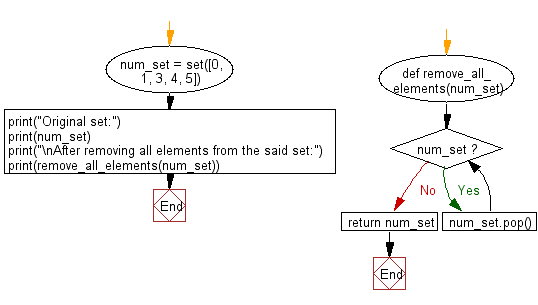﻿ Python: Remove items from Python set - w3resource# Python: Remove items from Python set

## Python sets: Exercise-4 with Solution

Write a Python program to remove item(s) from a given set.

Sample Solution-1:

Python Code:

Remove single element from a given set:

``````num_set = set([0, 1, 3, 4, 5])
print("Original set:")
print(num_set)
num_set.pop()
print("\nAfter removing the first element from the said set:")
print(num_set)
```
```

Sample Output:

```Original set:
{0, 1, 3, 4, 5}

After removing the first element from the said set:
{1, 3, 4, 5}
```

Pictorial Presentation:## Visualize Python code execution:

The following tool visualize what the computer is doing step-by-step as it executes the said program:

Sample Solution-2:

Python Code:

Remove all elements from a given set:

``````def remove_all_elements(num_set):
while num_set:
num_set.pop()
return num_set
num_set = set([0, 1, 3, 4, 5])
print("Original set:")
print(num_set)
print("\nAfter removing all elements from the said set:")
print(remove_all_elements(num_set))
```
```

Sample Output:

```Original set:
{0, 1, 3, 4, 5}

After removing all elements from the said set:
set()
```

Flowchart:## Visualize Python code execution:

The following tool visualize what the computer is doing step-by-step as it executes the said program:

Python Code Editor:

Have another way to solve this solution? Contribute your code (and comments) through Disqus.

What is the difficulty level of this exercise?

Test your Python skills with w3resource's quiz

﻿

## Python: Tips of the Day

While-else construct:

```i = 5

while i > 1:
print("Whil-ing away!")
i -= 1
if i == 3:
break
else:
print("Finished up!")
```